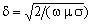# Mesh constraints

## Introduction

The mesh of the regions of the device, which are common to the two problems, must be adapted to the magnetic and thermal studied phenomena.

## Mesh constraint of the SM problem

The presence of the skin effect of the induced currents is well known. The area where eddy currents are non-negligible values should be finely meshed: there must be minimum two elements on the penetration depth.

Attention:

When the temperature increases, the magnetic permeability diminishes, and consequently the value of the penetration depthincreases. Thus, the mesh must be predicted accordingly.

## Mesh constraint of the TT problem

In the thermal problem, two phenomena cause exponential variations of the state variable that is the temperature, similar to the variation of electromagnetic quantities in case of the skin effect:

• the thermal conduction in the regions with high value of the temperature gradient, i.e. in thermal insulators. Pay attention, these are regions where we have the tendency to carry out a roughly mesh for the magnetic problem, because generally the thermal insulators are non-electroconductive regions;
• the heat transient distribution due to the thermal properties, specific heat and thermal conductivity. This is the case when the induced power density, which is the heat source, is completely placed at the surface of the part to be heated.

The thermal penetration depth is defined by the Peclet number (ratio between the transport term and the storage term of thermal equation). Pay attention, this quantity has sometimes “thinner” values than the penetration depth of induced currents.